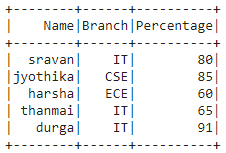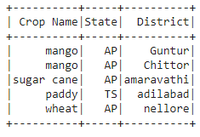# Create PySpark DataFrame from list of tuples

• Last Updated : 30 May, 2021

In this article, we are going to discuss the creation of a Pyspark dataframe from a list of tuples.

To do this, we will use the createDataFrame() method from pyspark. This method creates a dataframe from RDD, list or Pandas Dataframe. Here data will be the list of tuples and columns will be a list of column names.

Attention geek! Strengthen your foundations with the Python Programming Foundation Course and learn the basics.

To begin with, your interview preparations Enhance your Data Structures concepts with the Python DS Course. And to begin with your Machine Learning Journey, join the Machine Learning - Basic Level Course

Syntax:

dataframe = spark.createDataFrame(data, columns)

Example 1:

## Python3

 `# importing module``import` `pyspark`` ` `# importing sparksession from ``# pyspark.sql module``from` `pyspark.sql ``import` `SparkSession`` ` `# creating sparksession and giving``# an app name``spark ``=` `SparkSession.builder.appName(``'sparkdf'``).getOrCreate()`` ` `# list of tuples of college data``data ``=` `[(``"sravan"``, ``"IT"``, ``80``),``        ``(``"jyothika"``, ``"CSE"``, ``85``),``        ``(``"harsha"``, ``"ECE"``, ``60``),``        ``(``"thanmai"``, ``"IT"``, ``65``),``        ``(``"durga"``, ``"IT"``, ``91``)]`` ` `# giving column names of dataframe``columns ``=` `[``"Name"``, ``"Branch"``, ``"Percentage"``]`` ` `# creating a dataframe``dataframe ``=` `spark.createDataFrame(data, columns)`` ` `# show data frame``dataframe.show()`

Output:Example 2:

## Python3

 `# importing module``import` `pyspark`` ` `# importing sparksession from ``# pyspark.sql module``from` `pyspark.sql ``import` `SparkSession`` ` `# creating sparksession and giving ``# an app name``spark ``=` `SparkSession.builder.appName(``'sparkdf'``).getOrCreate()`` ` `# list of tuples of plants data``data ``=` `[(``"mango"``, ``"AP"``, ``"Guntur"``),``        ``(``"mango"``, ``"AP"``, ``"Chittor"``),``        ``(``"sugar cane"``, ``"AP"``, ``"amaravathi"``),``        ``(``"paddy"``, ``"TS"``, ``"adilabad"``),``        ``(``"wheat"``, ``"AP"``, ``"nellore"``)]`` ` `# giving column names of dataframe``columns ``=` `[``"Crop Name"``, ``"State"``, ``"District"``]`` ` `# creating a dataframe``dataframe ``=` `spark.createDataFrame(data, columns)`` ` `# show data frame``dataframe.show()`

Output:Example 3:

Python code to count the records (tuples) in the list

## Python3

 `# importing module``import` `pyspark`` ` `# importing sparksession from``# pyspark.sql module``from` `pyspark.sql ``import` `SparkSession`` ` `# creating sparksession and giving``# an app name``spark ``=` `SparkSession.builder.appName(``'sparkdf'``).getOrCreate()`` ` `#list of tuples of plants data``data ``=` `[(``"mango"``, ``"AP"``, ``"Guntur"``),``        ``(``"mango"``, ``"AP"``, ``"Chittor"``),``        ``(``"sugar cane"``, ``"AP"``, ``"amaravathi"``),``        ``(``"paddy"``, ``"TS"``, ``"adilabad"``),``        ``(``"wheat"``, ``"AP"``, ``"nellore"``)]`` ` `# giving column names of dataframe``columns ``=` `[``"Crop Name"``, ``"State"``, ``"District"``]`` ` `# creating a dataframe ``dataframe ``=` `spark.createDataFrame(data, columns)`` ` `#count records in the list``dataframe.count()`

Output:

`5`

My Personal Notes arrow_drop_up